Electron. J. Differential Equations, Vol. 2019 (2019), No. 62, pp. 1-11.

### Asymptotic formulas for oscillatory bifurcation diagrams of semilinear ordinary differential equations Tetsutaro Shibata

Abstract:
We study the nonlinear eigenvalue problem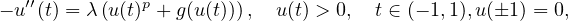where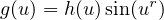,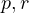are given constants satisfying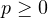,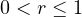and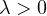is a parameter. It is known that under suitable conditions on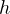,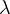is parameterized by the maximum norm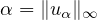of the solution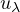associated withand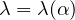is a continuous function for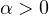. When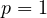,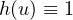and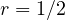, this equation has been introduced by Chen  as a model equation such that there exist infinitely many solutions near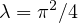. We prove thatis an oscillatory bifurcation curve as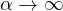by showing the asymptotic formula for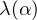. It is found that the shapes of bifurcation curves depend on the condition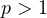or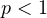. time-map argument and stationary phase method.

Submitted October 31, 2018. Published May 7, 2019.
Math Subject Classifications: 34C23, 34F10.
Key Words: Oscillatory bifurcation; time-map argument; stationary phase method.

Show me the PDF file (333 KB), TEX file for this article.Tetsutaro Shibata Laboratory of Mathematics Graduate School of Engineering Hiroshima University Higashi-Hiroshima, 739-8527, Japan email: tshibata@hiroshima-u.ac.jp